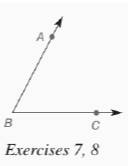Chapter 7.CR, Problem 7CRElementary Geometry For College St...

7th Edition
Alexander + 2 others
ISBN: 9781337614085

Solutions

Chapter
SectionElementary Geometry For College St...

7th Edition
Alexander + 2 others
ISBN: 9781337614085
Textbook Problem

In Review Exercises 7 to 13, sketch and describe the locus in a plane.Find the locus of points equidistant from the sides of ∠ A B C .To determine

To find: The locus of points equidistant from the sides of ABC.

Explanation

Given:

The ABC is shown below

Theorem used:

The locus of points in a plane and equidistant from the sides of an angle is the angle bisector.

Calculation:

Consider the given figure

By the theorem, we get the locus as the angle bisector as shown below

From, the figure we see that BD bisects ABC

Still sussing out bartleby?

Check out a sample textbook solution.

See a sample solution

The Solution to Your Study Problems

Bartleby provides explanations to thousands of textbook problems written by our experts, many with advanced degrees!

Get Started

Evaluate the integrals in Problems 5-28. Check your answers by differentiating. 16.

Mathematical Applications for the Management, Life, and Social Sciences

Calculate y'. 24. y=1/x+x3

Single Variable Calculus: Early Transcendentals, Volume I

f(x) = x3 2x2 + x 1 has a local maximum at: a) 0 b) 1 c) 13 d) f has no local maxima

Study Guide for Stewart's Single Variable Calculus: Early Transcendentals, 8th